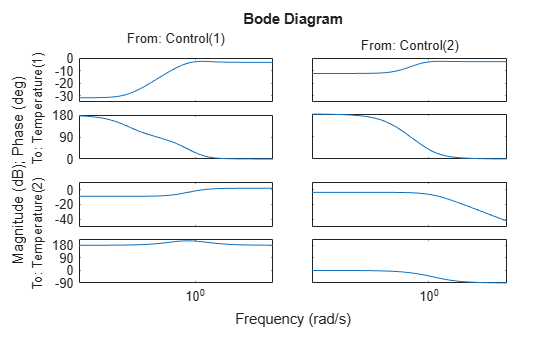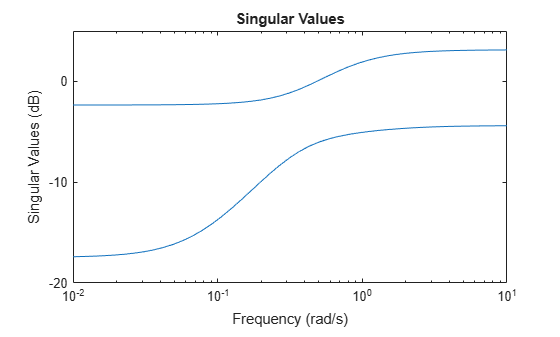# Frequency Response of a MIMO System

This example shows how to examine the frequency response of a multi-input, multi-output (MIMO) system in two ways: by computing the frequency response, and by computing the singular values.

Calculate the frequency response of a MIMO model and examine the size of the output.

```H = rss(2,2,2); H.InputName = 'Control'; H.OutputName = 'Temperature'; [mag,phase,w] = bode(H); size(mag)```
```ans = 1×3 2 2 70 ```

The first and second dimension of the data array `mag` are the number of outputs and inputs of `H`. The third dimension is the number of points in the frequency vector `w`. (The `bode` command determines this number automatically if you do not supply a frequency vector.) Thus, `mag(i,j,:)` is the frequency response from the `j` th input of `H` to the `i` th output, in absolute units. The phase data array `phase` takes the same form as `mag`.

Plot the frequency response of each input/output pair in `H`.

`bode(H)``bode` plots the magnitude and the phase of the frequency response of each input/output pair in `H`. (Because `rss` generates a random state-space model, you might see different responses from those pictured.) The first column of plots shows the response from the first input, `Control(1)`, to each output. The second column shows the response from the second input, `Control(2)`, to each output.

Plot the singular values of `H` as a function of frequency.

`sigma(H)``sigma` plots the singular values of the MIMO system `H` as a function of frequency. The maximum singular value at a particular frequency is the maximum gain of the system over all linear combinations of inputs at that frequency. Singular values can provide a better indication of the overall response, stability, and conditioning of a MIMO system than a channel-by-channel Bode plot.

Calculate the singular values of `H` between 0.1 and 10 rad/s.

`[sv,w] = sigma(H,{0.1,10});`

When you call `sigma` with output arguments, the command returns the singular values in the data array `sv`. The cell array input `{0.1,10}` tells `sigma` to calculate the singular values at a grid of frequencies between 0.1 and 10 rad/s. `sigma` returns these frequencies in the vector `w`. Each row of `sv` contains the singular values of `H` at the frequencies of `w`.